# 数据结构—八大排序

本文所有排序以升序为例子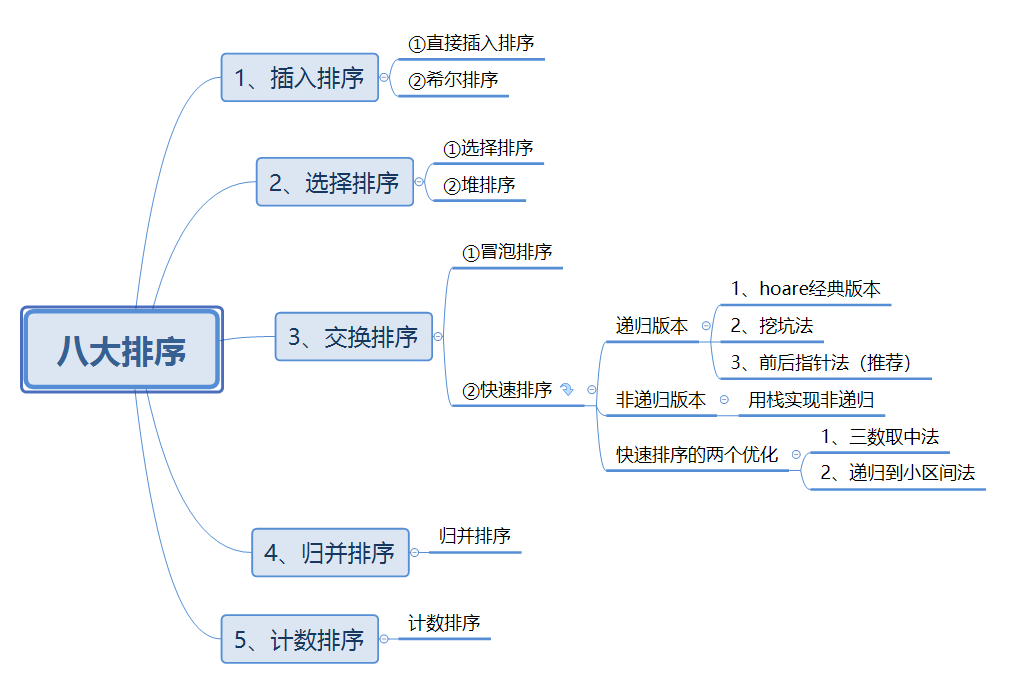1、hoare版本

2、挖坑法

3、前后指针法（推荐这种写法）

1、三数取中法

2、递归到小子区间

# 一、直接插入排序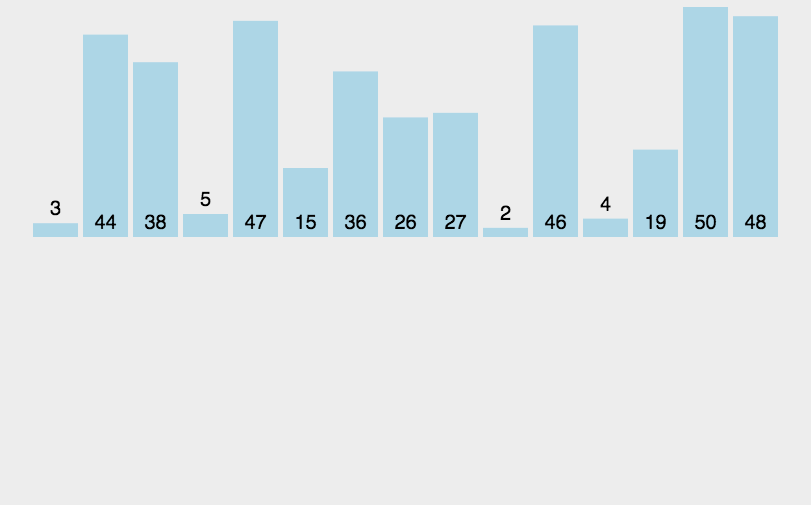1、当插入第n个元素时，前面的n-1个数已经有序

2、用这第n个数与前面的n-1个数比较，找到要插入的位置，将其插入（原来位置上的数不会被覆盖，因为提前保存了）

3、原来位置上的数据，依次后移

具体实现：

①单趟的实现（将x插入到 [0,end] 的有序区间）

（1）待插入的数字是在前面有序数字的中间数，直接比较将x赋值给end+1位置

（2）x是最小的一个数，end就会到达-1的位置，最后直接将x赋值给end+1位置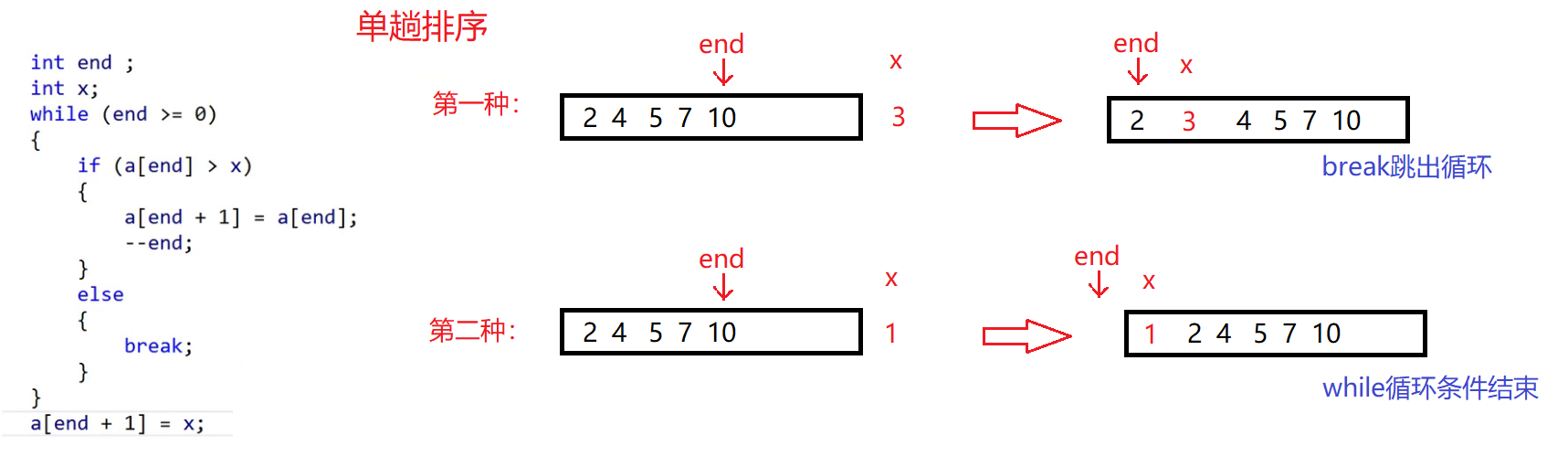②整个数组排序的实现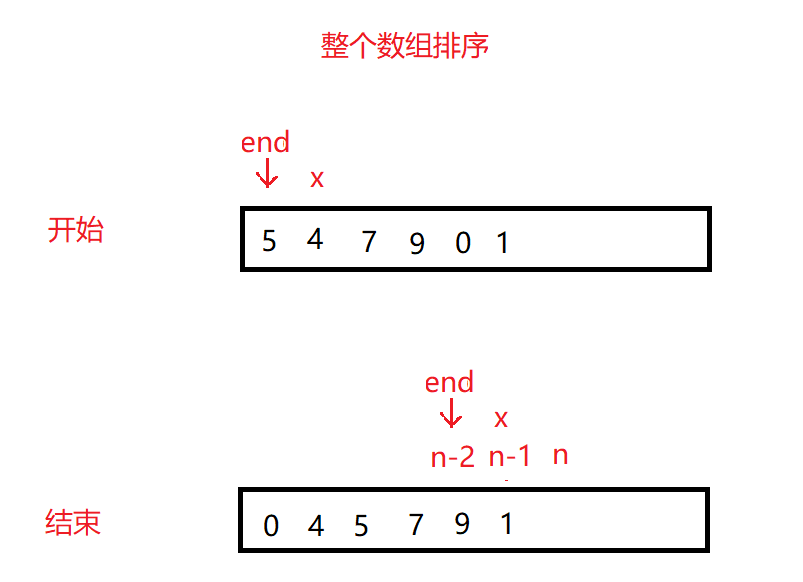总体代码：

void InsertSort(int* a, int n)
{
assert(a);

for (int i = 0; i < n - 1; ++i)
{
int end = i;
int x=a[end+1];//将end后面的值保存到x里面了
//将x插入到[0，end]的有序区间
while (end >= 0)
{
if (a[end] > x)
{
a[end + 1] = a[end];  //往后挪动一位
--end;
}
else
{
break;
}
}
a[end + 1] = x;      //x放的位置都是end的后一个位置
}

}

①元素越接近有序，直接插入排序的效率越高

②时间复杂度:O(N^2)

③空间复杂度：O(1)

# 二、希尔排序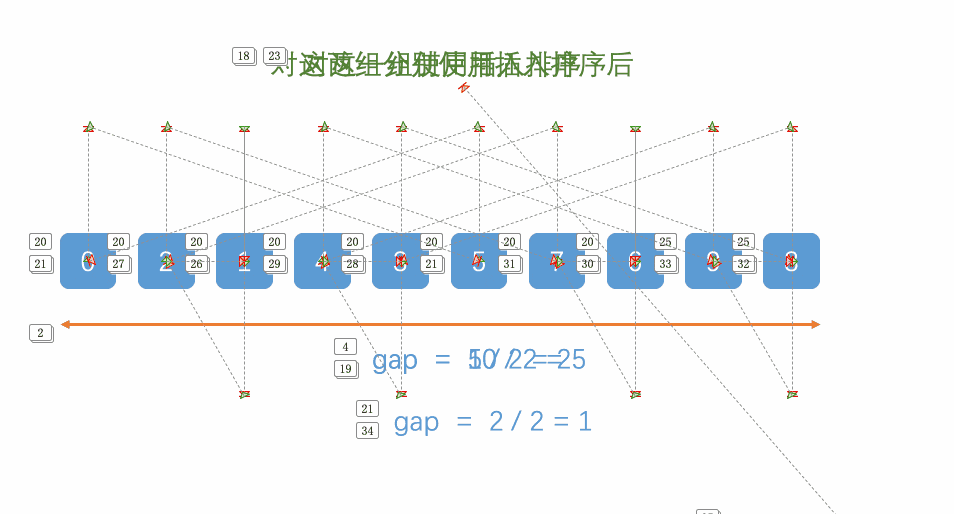1、先选定个小于n的数字作为gap，所有距离为gap的数分为一组进行预排序（直接插入排序）

2、再选一个小于gap的数，重复①的操作

3、当gap=1时，相当于整个数组就是一组，再进行一次插入排序即可整体有序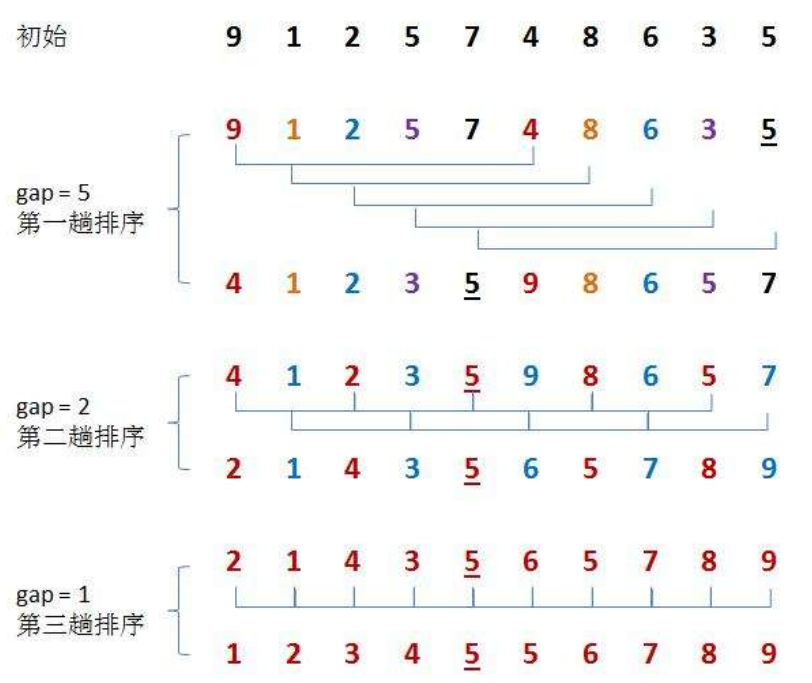①单组排序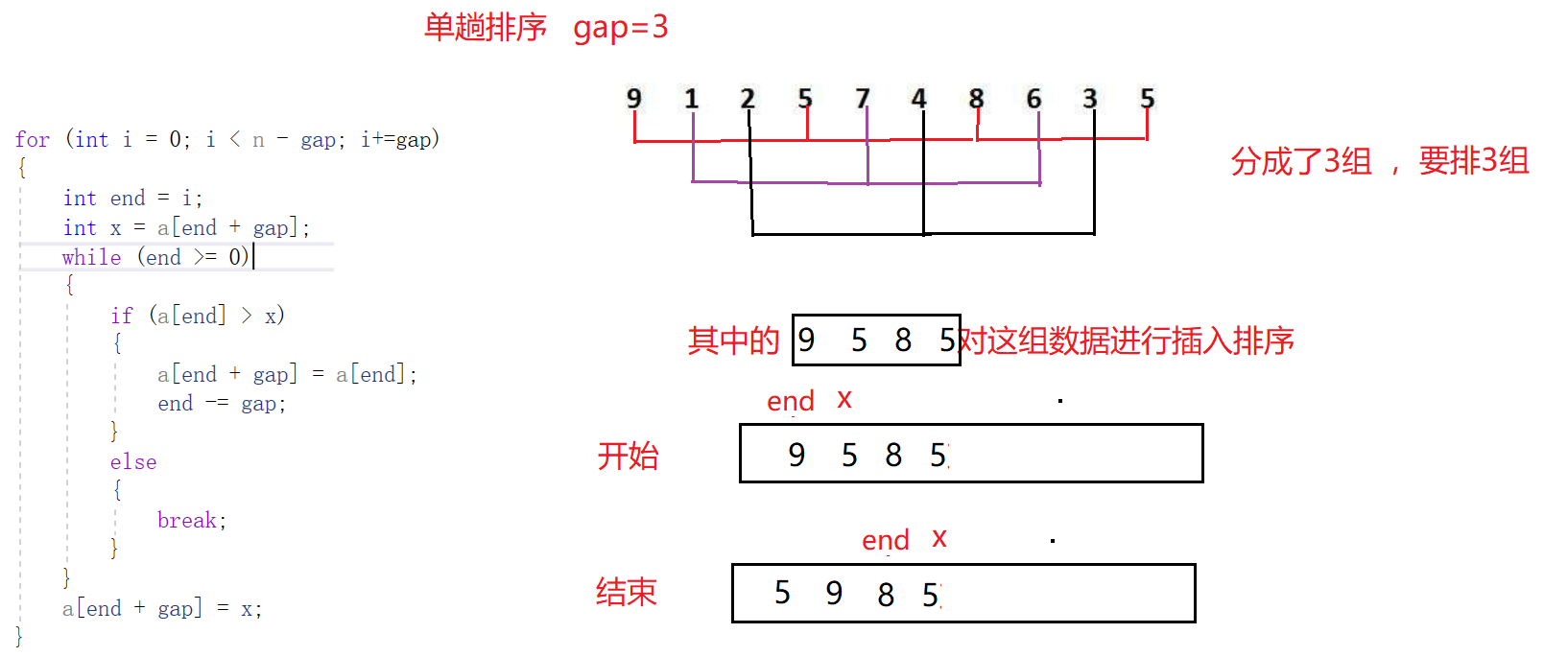②多组进行排序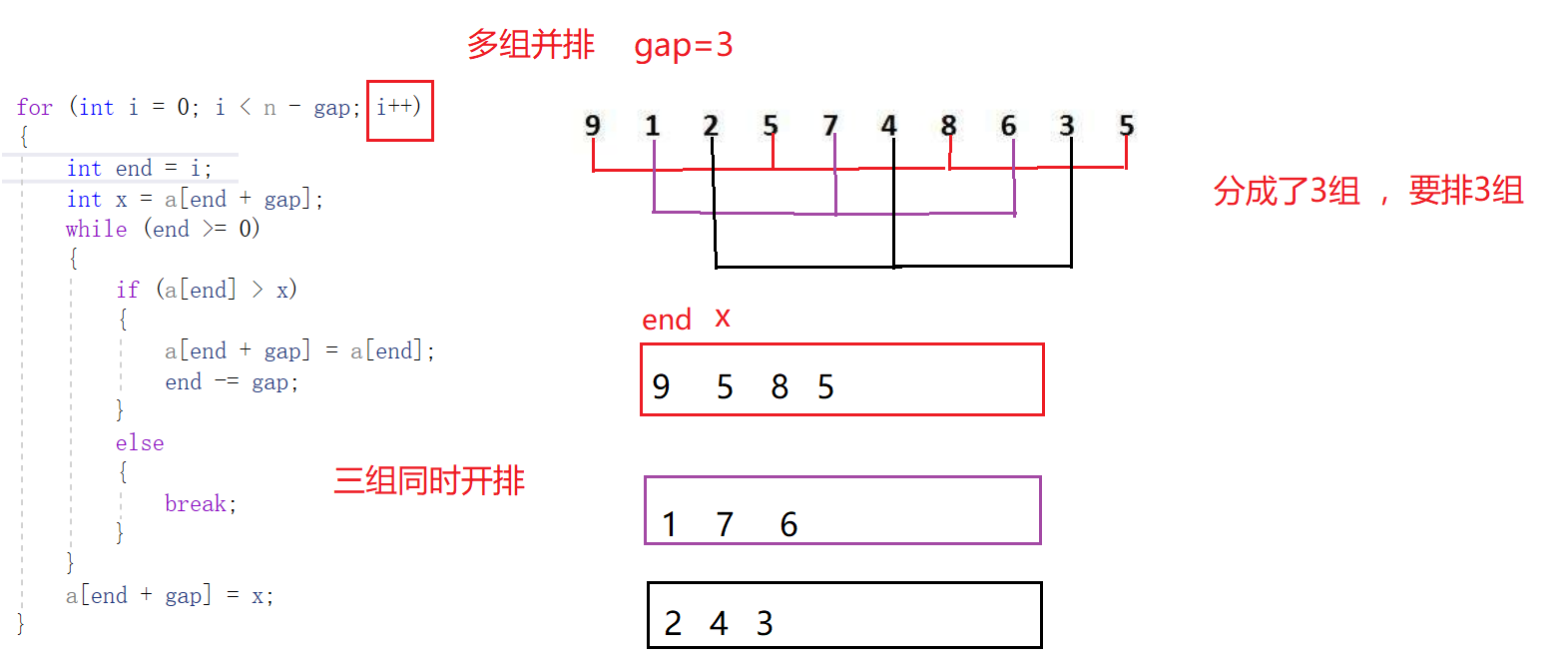③整个数组进行排序（控制gap）

（1）gap越大，预排越快，越不接近于有序

（2）gap越小，预排越慢，越接近有序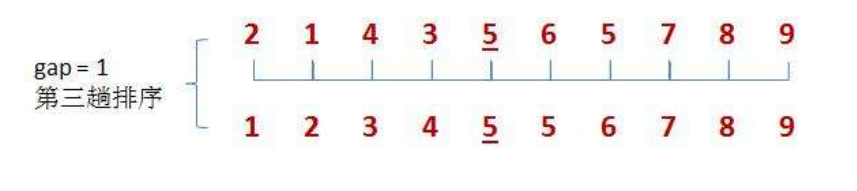void ShellSort(int* a, int n)
{

int gap = n;
while (gap > 1)
{
gap /= 2;

for (int i = 0; i < n - gap; i++)
{
int end = i;
int x = a[end + gap];
while (end >= 0)
{
if (a[end] > x)
{
a[end + gap] = a[end];
end -= gap;
}
else
{
break;
}
}
a[end + gap] = x;
}
}
}

①希尔排序是对直接插入排序的优化

②时间复杂度：O(N^1.3)

③空间复杂度：O(1)

# 三、选择排序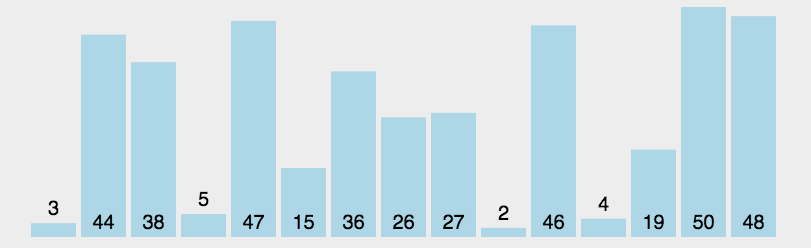①单趟排序

ps：其中的begin，end保存记录左右的下标，mini，maxi记录保存最小值和最大值得下标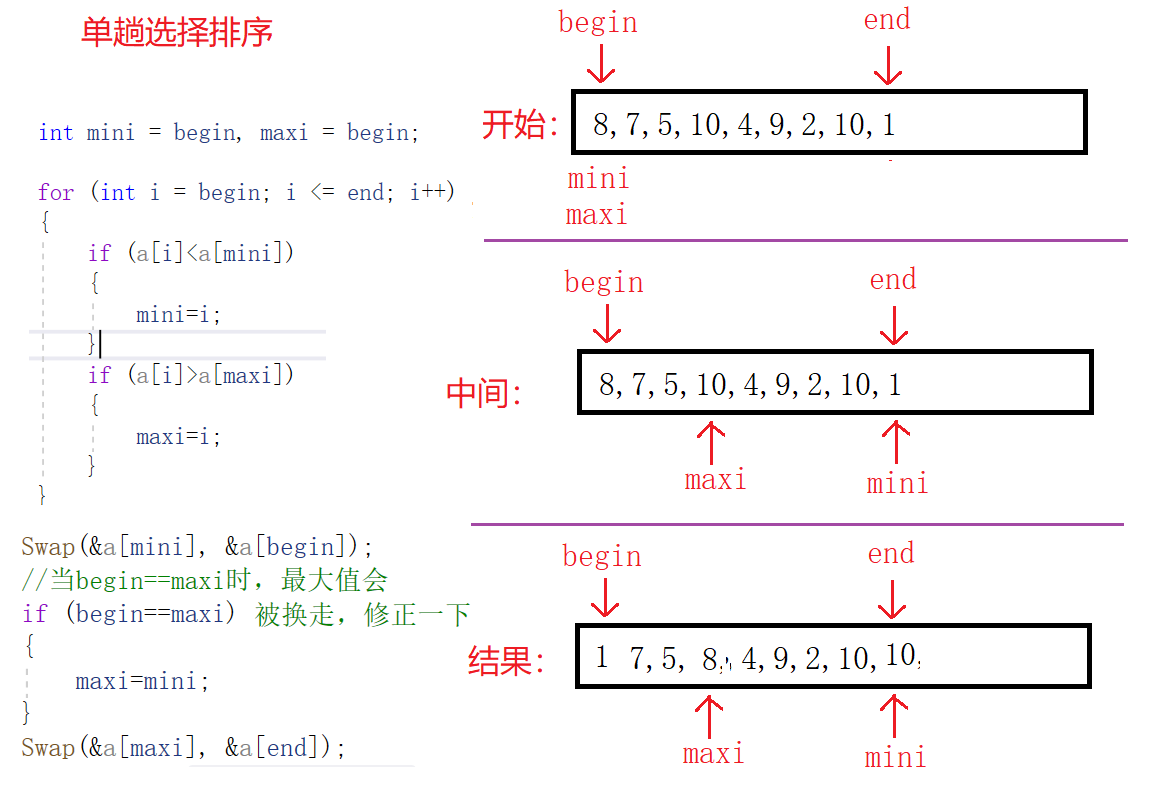②整个数组排序

begin++和end--这样下次就可以排剩下的n-2个数字，再次进行单趟，如此可构成循环，直到begin小于end

void SelectSort(int* a, int n)
{
int begin = 0,end = n - 1;

while (begin<end)
{
int mini = begin, maxi = begin;

for (int i = begin; i <= end; i++)
{
if (a[i] < a[mini])
{
mini = i;
}
if (a[i] > a[maxi])
{
maxi = i;
}
}
Swap(&a[mini], &a[begin]);
//当begin==maxi时，最大值会被换走，修正一下
if (begin==maxi)
{
maxi=mini;
}
Swap(&a[maxi], &a[end]);
begin++;
end--;
}
}

①直接选择排序很好理解，但实际效率不高，很少使用

②时间复杂度：O(N^2)

③空间复杂度：O(1)

# 四、堆排序

1、将待排序的序列构造成一个大堆，根据大堆的性质，当前堆的根节点（堆顶）就是序列中最大的元素；

2、将堆顶元素和最后一个元素交换，然后将剩下的节点重新构造成一个大堆；

3、重复步骤2，如此反复，从第一次构建大堆开始，每一次构建，我们都能获得一个序列的最大值，然后把它放到大堆的尾部。最后，就得到一个有序的序列了。

①向下调整算法

（1）若想将其调整为小堆，那么根结点的左右子树必须都为小堆。
（2）若想将其调整为大堆，那么根结点的左右子树必须都为大堆。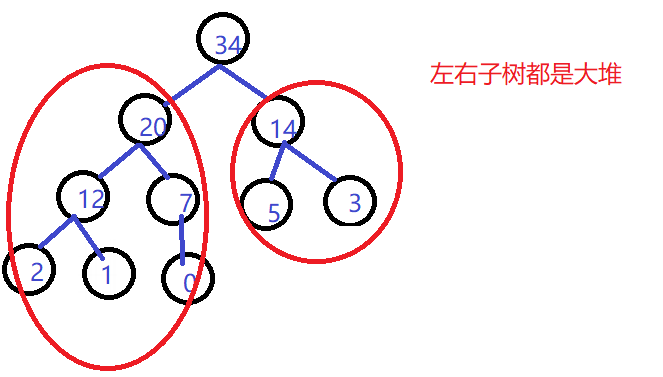1、从根节点开始，选出左右孩子值较大的一个

2、如果选出的孩子的值大于父亲的值，那么就交换两者的值

3、将大的孩子看做新的父亲，继续向下调整，直到调整到叶子节点为止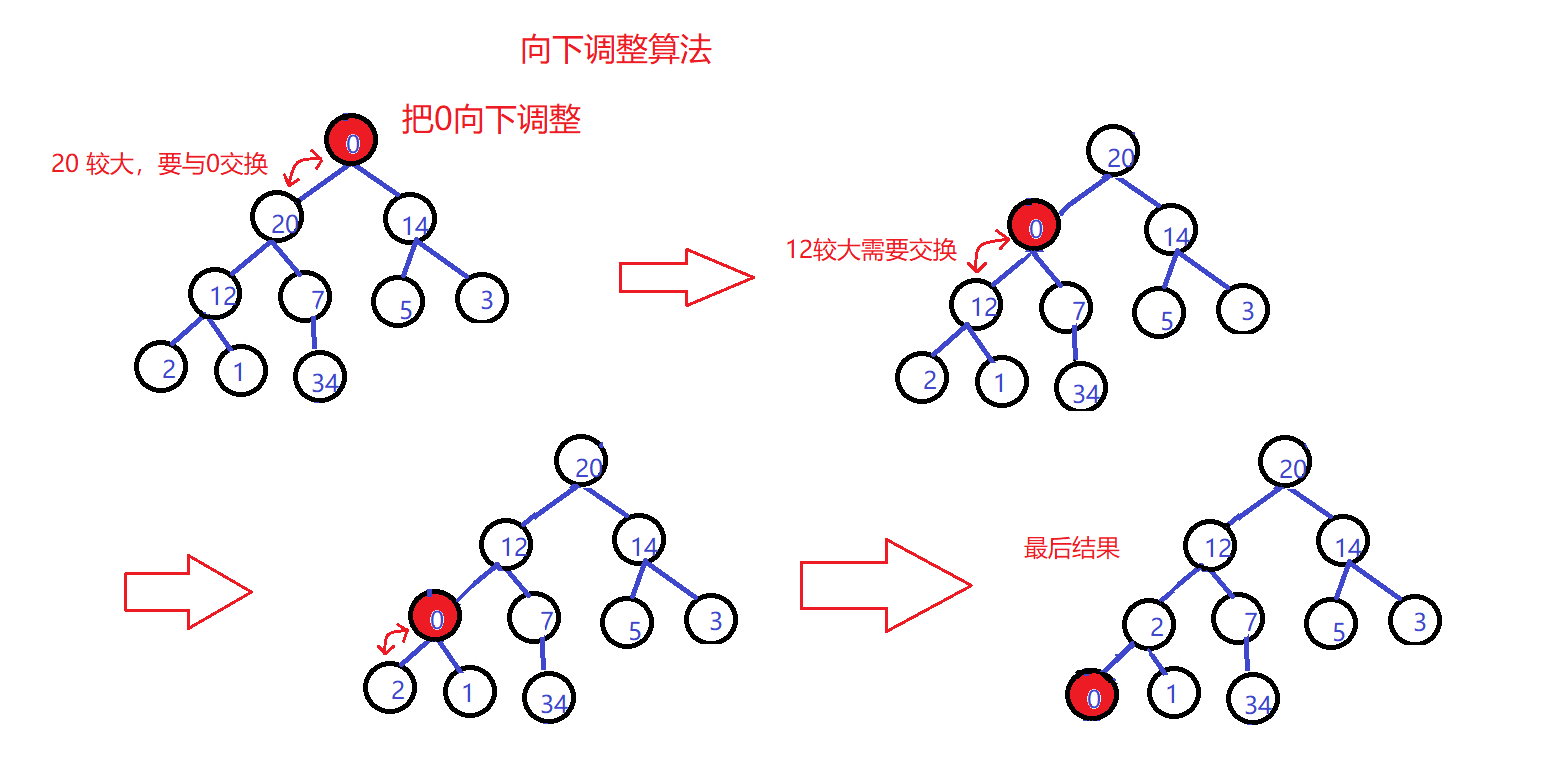//向下调整算法
//以建大堆为例
void AdJustDown(int* a, int n, int parent)
{
int child = parent * 2 + 1;
//默认左孩子较大
while (child < n)
{
if (child + 1 < n && a[child+1] > a[child ])//如果这里右孩子存在，
//且更大，那么默认较大的孩子就改为右孩子
{
child++;
}
if(a[child]>a[parent])
{
Swap(&a[child], &a[parent]);
parent = child;
child = parent * 2 + 1;
}
else
{
break;
}
}
}

②建堆（将给定的任意数组建成大堆）

建堆图示：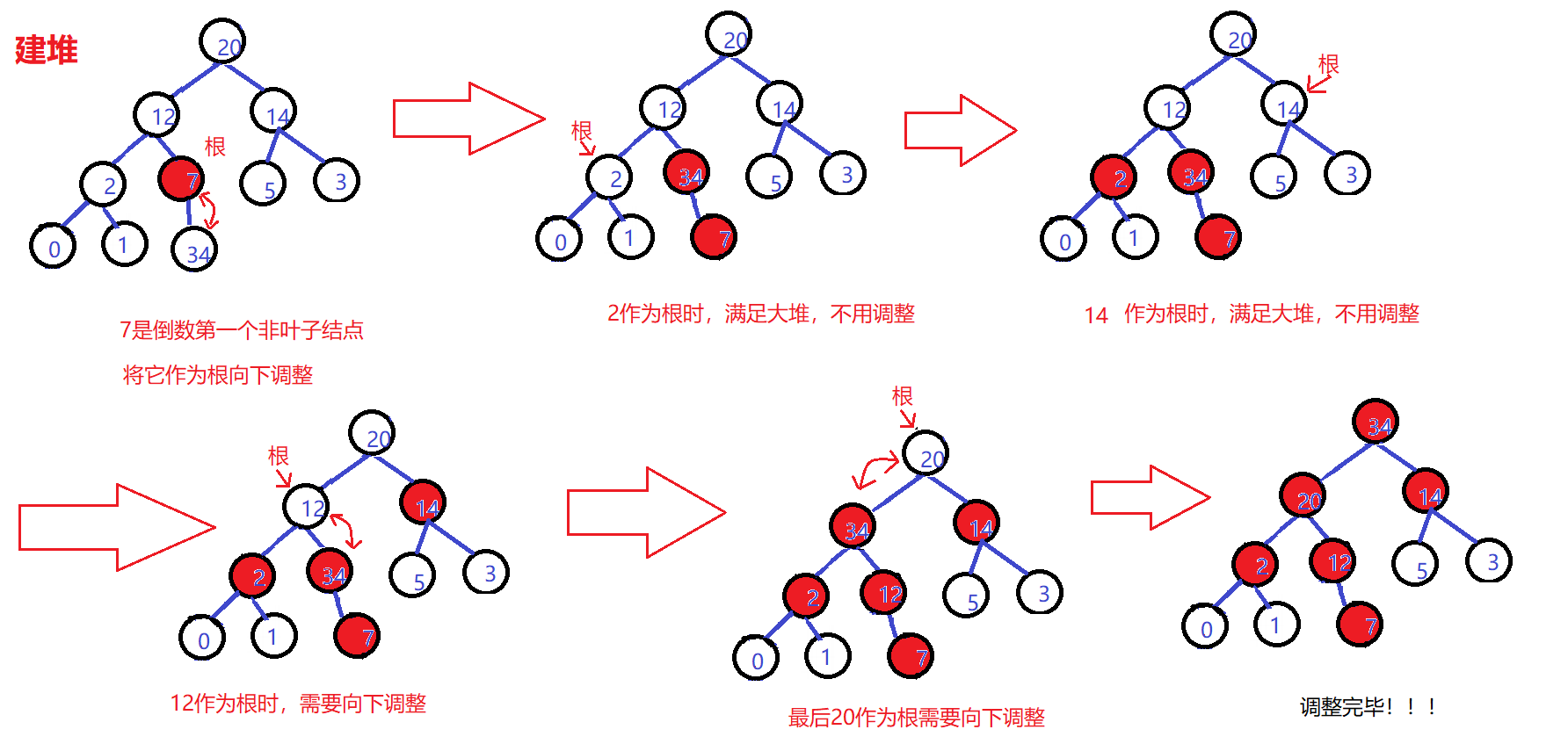//最后一个叶子结点的父亲为i，从后往前，依次向下调整，直到调到根的位置
for (int i = (n - 1 - 1) / 2; i >= 0; --i)
{
}

③堆排序（利用堆删的思想进行）

1、建好堆之后，将堆顶的数字与最后一个数字交换
2、将最后一个数字不看，剩下的n-1个数字再向下调整成堆再进行第1步

3、直到最后只剩一个数停止，这样就排成有序的了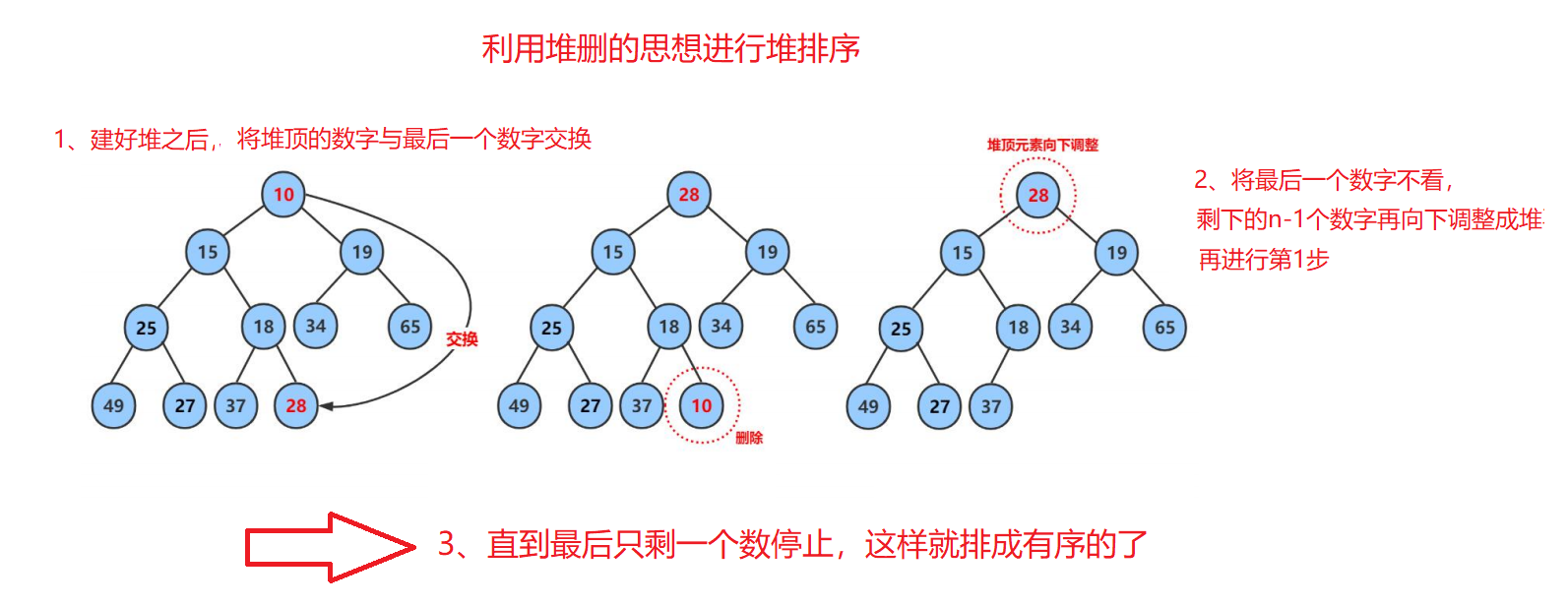for (int end = n - 1; end > 0; --end)
{
Swap(&a[end], &a);
}

void AdJustDown(int* a, int n, int parent)
{
int child = parent * 2 + 1;

while (child < n)
{
if (child + 1 < n && a[child+1] > a[child ])

{
child++;
}
if(a[child]>a[parent])
{
Swap(&a[child], &a[parent]);
parent = child;
child = parent * 2 + 1;
}
else
{
break;
}
}
}

//堆排序
void HeapSort(int*a,int n)
{

for (int i = (n - 1 - 1) / 2;i>=0;--i)
{
}

for (int end = n - 1; end > 0; --end)
{
Swap(&a[end],&a);
}
}

# 五、冒泡排序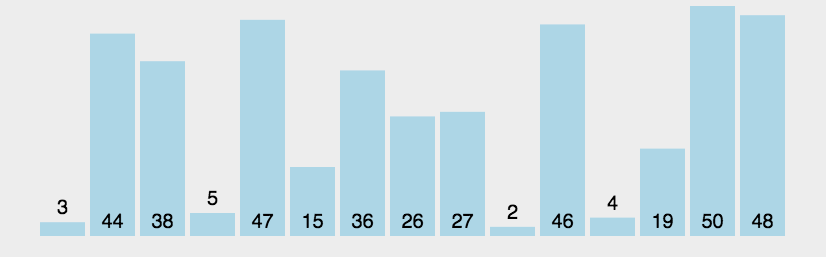//优化版本的冒泡排序
void BubbleSort(int* a, int n)
{
int end = n-1;
while (end>0)
{
int exchange = 0;
for (int i = 0; i < end; i++)
{
if (a[i] > a[i + 1])
{
Swap(&a[i], &a[i + 1]);
exchange = 1;
}
}
if (exchange == 0)//单趟过程中，若没有交换过，证明已经有序，没有必要再排序
{
break;
}
end--;
}
}

冒泡排序总结：

①非常容易理解的排序

②时间复杂度:O(N^2)

③空间复杂度:O(1)

# 六、快速排序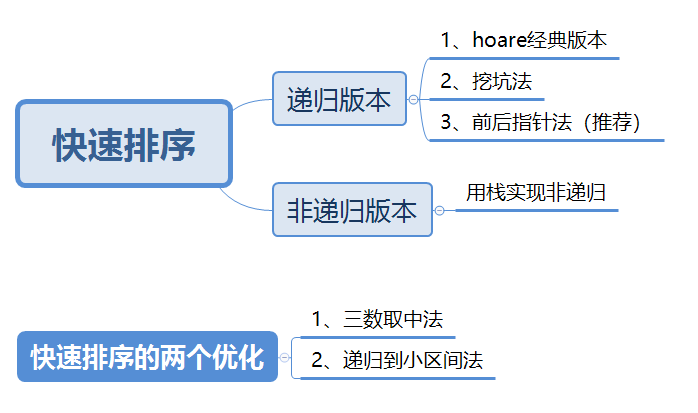## 递归版本

### 1、hoare版本

hoare的单趟思想：

1、左边作key，右边先走找到比key小的值

2、左边后走找到大于key的值

3、然后交换left和right的值

4、一直循环重复上述1 2 3步

5、两者相遇时的位置，与最左边选定的key值交换

//hoare版本
//单趟排序  让key到正确的位置上   keyi表示key的下标，并不是该位置的值
int partion1(int* a, int left, int right)
{
int keyi = left;//左边作keyi
while (left < right)
{   //右边先走，找小于keyi的值
while (left < right && a[right] >= a[keyi])
{
right--;
}
//左边后走，找大于keyi的值
while (left < right && a[left] <= a[keyi])
{
left++;
}
Swap(&a[left], &a[right]);
}
Swap(&a[left], &a[keyi]);
return left;
}

void QuickSort(int* a, int left, int right)
{
if (left >= right)
return;

int keyi = partion1(a, left, right);
//[left,keyi-1] keyi [keyi+1,right]
QuickSort(a, left, keyi - 1);
QuickSort(a, keyi + 1, right);
}

### 2、挖坑法

1、先将最左边第一个数据存放在临时变量key中，形成一个坑位

2、右边先出发找到小于key的值，然后将该值丢到坑中去，此时形成一个新坑位

3、左边后出发找到大于key的值，将该值丢入坑中去，此时又形成一个新的坑位

4、一直循环重复1 2 3步

5、直到两边相遇时，形成一个新的坑，最后将key值丢进去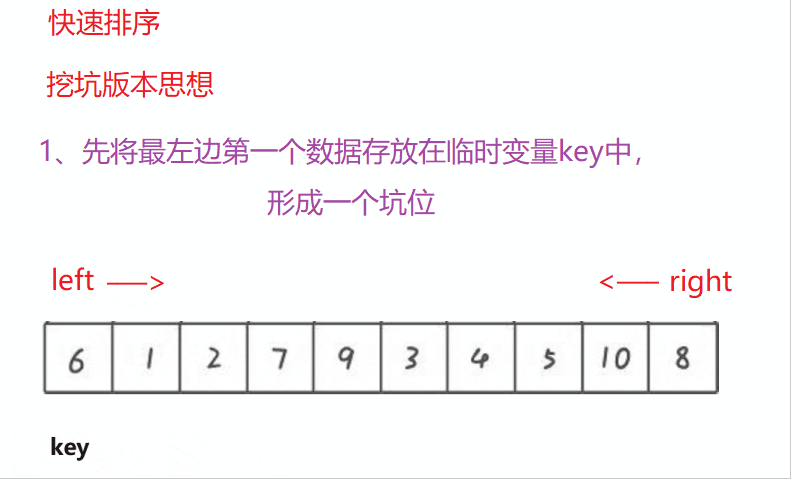//挖坑法
int partion2(int* a, int left, int right)
{
int key = a[left];
int pit = left;
while (left < right)
{
while (left < right && a[right] >= key)
{
right--;
}
a[pit] = a[right];//填坑
pit=right;

while (left < right && a[left] <= key)
{
left++;
}
a[pit] = a[left];//填坑
pit=left;
}
a[pit] = key;
return pit;
}

void QuickSort(int* a, int left, int right)
{
if (left >= right)
return;

int keyi = partion2(a, left, right);
//[left,keyi-1] keyi [keyi+1,right]
QuickSort(a, left, keyi - 1);
QuickSort(a, keyi + 1, right);
}

### 3、前后指针法（推荐这种写法）

1、初始时选定prev为序列的开始，cur指针指向prev的后一个位置，同样选择最左边的第一个数字作为key

2、cur先走，找到小于key的值，找到就停下来

3、++prev

4、交换prev和cur为下标的值

5、一直循环重复2 3 4步，停下来后，最后交换key和prev为下标的值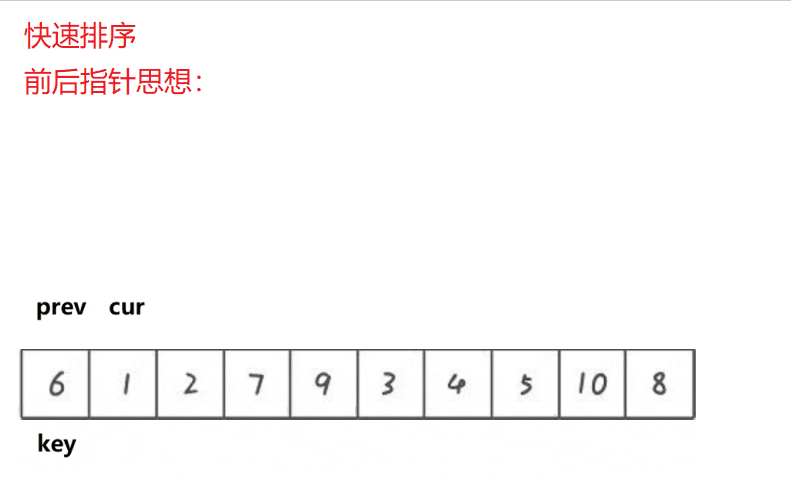int partion3(int* a, int left, int right)
{
int prev = left;
int cur = left + 1;
int keyi = left;
while (cur <= right)
{
if (a[cur] < a[keyi] && ++prev != cur)//prev != cur  防止cur和prev相等时，相当于自己和自己交换，可以省略
{                                   //前置 ++ 的优先级大于 != 不等于的优先级
Swap(&a[prev], &a[cur]);
}
++cur;
}
Swap(&a[keyi], &a[prev]);
return prev;
}

void QuickSort(int* a, int left, int right)
{
if (left >= right)
return;

int keyi = partion3(a, left, right);
//[left,keyi-1] keyi [keyi+1,right]
QuickSort(a, left, keyi - 1);
QuickSort(a, keyi + 1, right);
}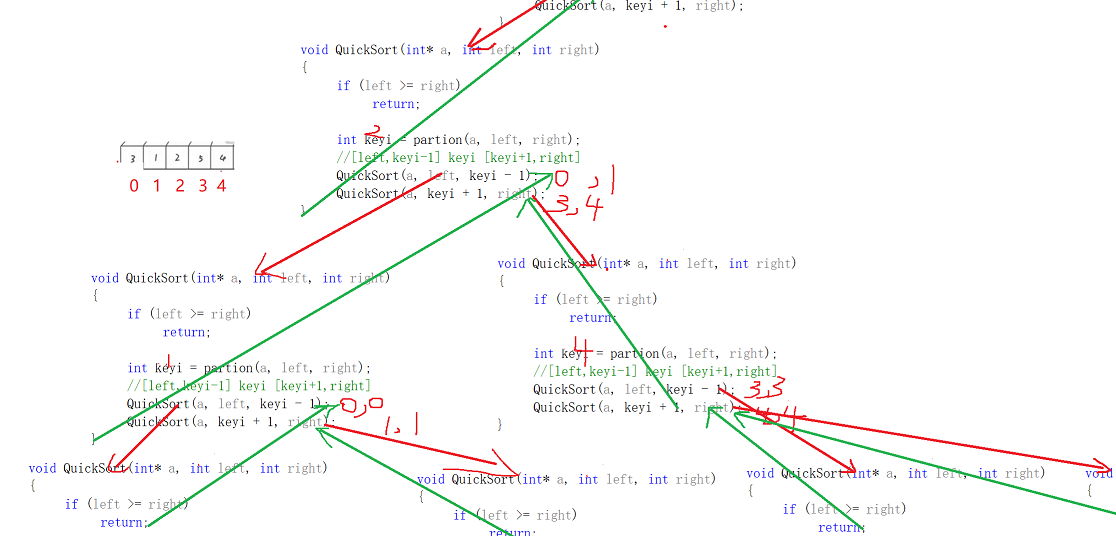## 快速排序的优化

### 1、三数取中法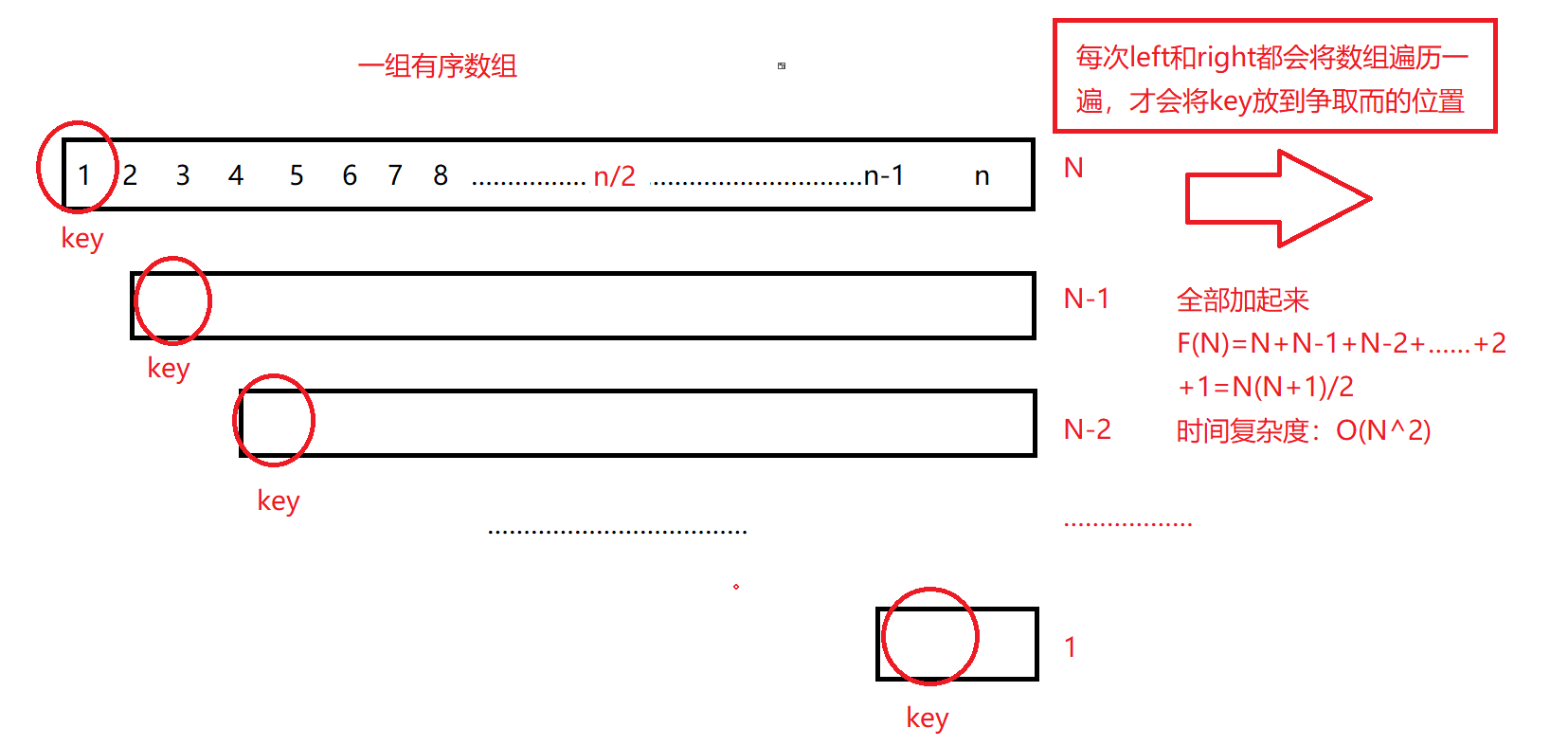//快排的优化
//三数取中法
int GetMidIndex(int* a, int left, int right)
{
int mid = (left + right) / 2;

if (a[left] < a[right])
{
if (a[mid] < a[right])
{
return mid;
}
else if (a[mid] > a[right])
{
return right;
}
else
{
return left;
}
}

else
{

if (a[mid] > a[left])
{
return left;
}
else if (a[mid] < a[right])
{
return right;
}
else
{
return mid;
}
}

}
int partion5(int* a, int left, int right)
{
//三数取中,面对有序时是最坏的情况O(N^2)，现在每次选的key都是中间值，变成最好的情况了
int midi = GetMidIndex(a, left, right);
Swap(&a[midi], &a[left]);//这样还是最左边作为key

int prev = left;
int cur = left + 1;
int keyi = left;
while (cur <= right)
{
if (a[cur] < a[keyi] && ++prev != cur)//prev != cur  防止cur和prev相等时，相当于自己和自己交换，可以省略
{                                   //前置 ++ 的优先级大于 != 不等于的优先级
//++prev;
Swap(&a[prev], &a[cur]);
}
++cur;
}
Swap(&a[keyi], &a[prev]);
return prev;
}

### 2、递归到小子区间

//小区间优化法，可以采用直接插入排序
void QuickSort(int* a, int left, int right)
{
if (left >= right)
return;

if (right - left + 1 < 10)
{
InsertSort(a + left, right - left + 1);
}
else
{
int keyi = partion5(a, left, right);
//[left,keyi-1] keyi [keyi+1,right]
QuickSort(a, left, keyi - 1);
QuickSort(a, keyi + 1, right);
}
}

## 非递归版本

1. 申请一个栈，存放排序数组的起始位置和终点位置。

2. 将整个数组的起始位置和终点位置入栈。

3. 由于栈的特性是：后进先出，right后进栈，所以right先出栈。

4. 对数组进行一次单趟排序，返回key关键值的下标。

5. 这时候需要排基准值key左边的序列。

6.判断栈是否为空，若不为空 重复4、5步、若为空则排序完成。

void QuickSortNonR(int* a, int left, int right)
{
Stack st;
StackInit(&st);
StackPush(&st,left);
StackPush(&st, right);

while (!StackEmpty(&st))
{
int end = StackTop(&st);
StackPop(&st);

int begin = StackTop(&st);
StackPop(&st);

int keyi = partion5(a,begin,end);
//区间被成两部分了 [begin,keyi-1] keyi [keyi+1,end]
if (keyi + 1 < end)
{
StackPush(&st,keyi+1);
StackPush(&st,end);
}
if (keyi-1>begin)
{
StackPush(&st, begin);
StackPush(&st, keyi -1);
}
}
StackDestroy(&st);
}

①快排的整体综合性能和使用场景都是比较好的，所以才敢叫快速排序

②快排唯一死穴，就是排一些有序或者接近有序的序列，例如 2,3,2,3,2,3,2,3这样的序列时，会变成O(N^2)的时间复杂度

③时间复杂度O(N*logN)

④空间复杂度O(logN)

# 七、归并排序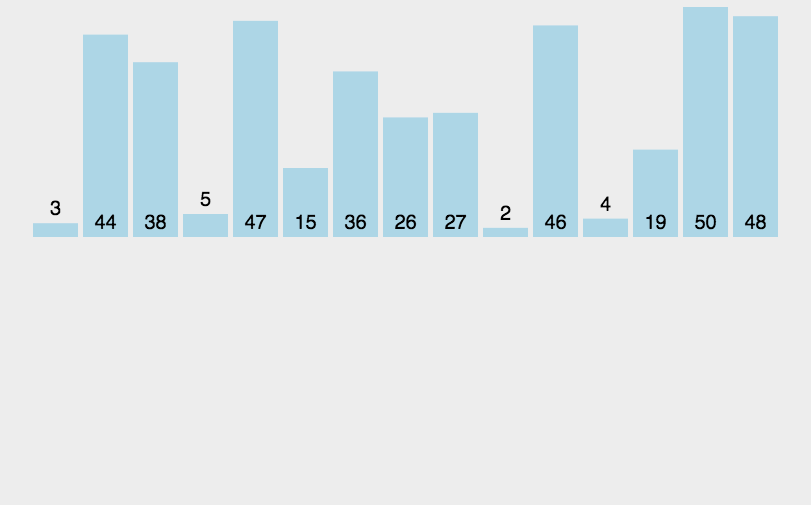归并排序的基本思想（分治思想）：

1、（拆分）将一段数组分为左序列和右序列，让他们两个分别有序，再将左序列细分为左序列和右序列，如此重复该步骤，直到细分到区间不存在或者只有一个数字为止

2、（合并）将第一步得到的数字合并成有序区间

具体实现：

①拆分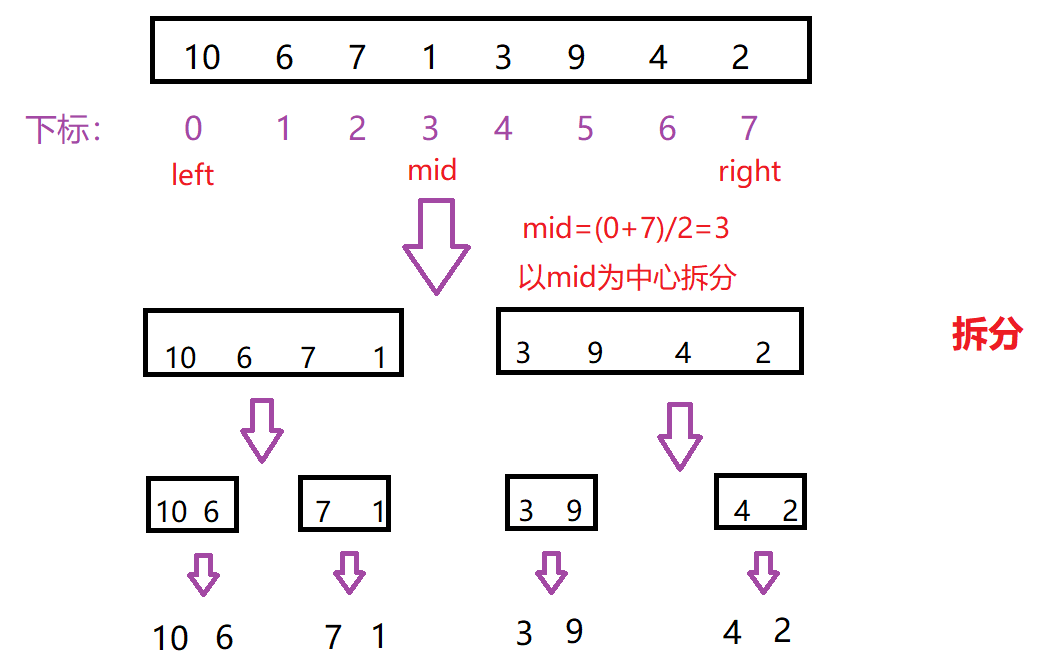②合并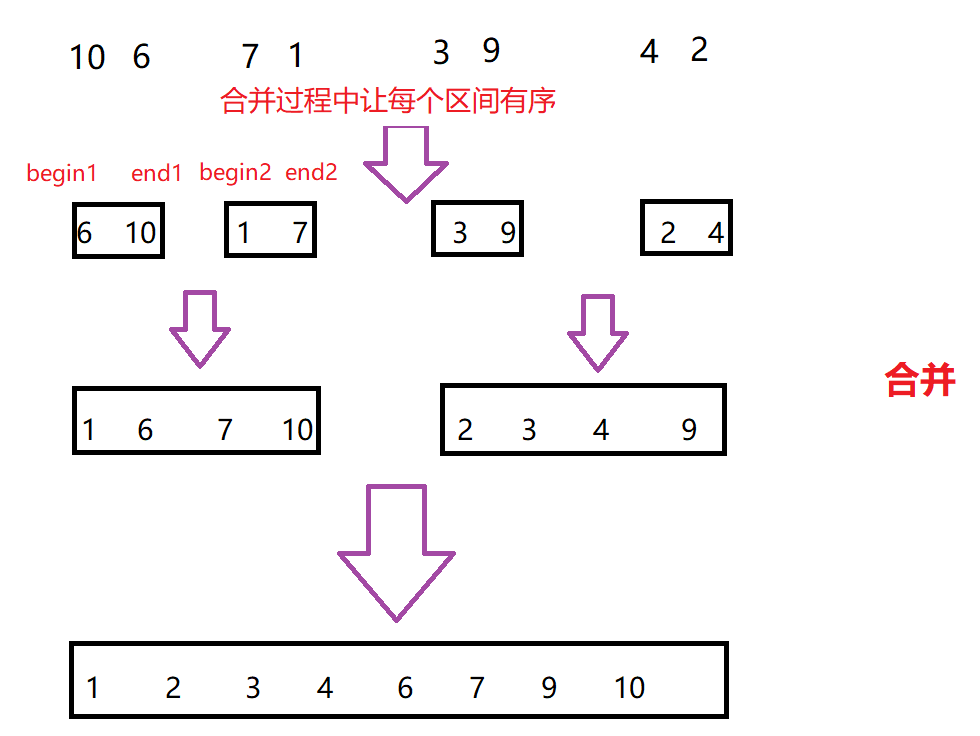## 递归实现：

void _MergeSort(int* a, int left, int right, int* tmp)
{
if (left >= right)
{
return;
}
int mid = (left + right) / 2;
_MergeSort(a, left, mid, tmp);
_MergeSort(a, mid+1, right, tmp);

int begin1 = left, end1 = mid;
int begin2 = mid + 1, end2 = right;
int i = left;
while (begin1 <= end1 && begin2 <= end2)
{
if (a[begin1] < a[begin2])
{
tmp[i++] = a[begin1++];
}
else
{
tmp[i++] = a[begin2++];
}
}
while (begin1 <= end1)
{
tmp[i++] = a[begin1++];
}
while (begin2 <= end2)
{
tmp[i++] = a[begin2++];
}
for (int j = left; j <= right; j++)
{
a[j] = tmp[j];
}
}
//归并排序
void MergeSort(int* a, int n)
{
int* tmp = (int*)malloc(sizeof(int)*n);
if (tmp == NULL)
{
printf("malloc fail\n");
exit(-1);
}
_MergeSort(a,0,n-1,tmp);

free(tmp);
tmp = NULL;
}

## 非递归实现：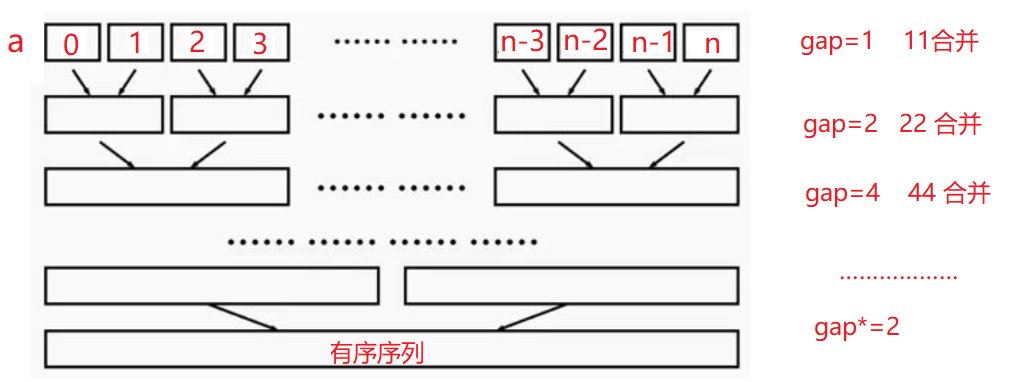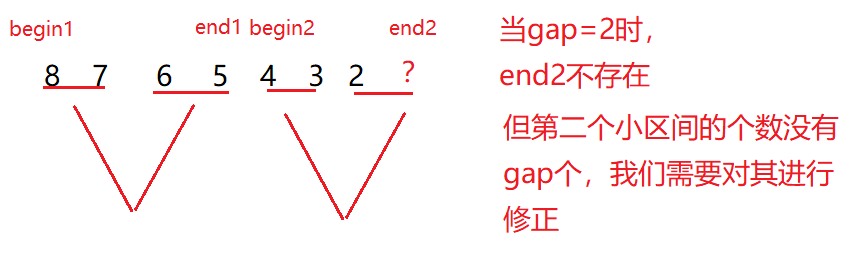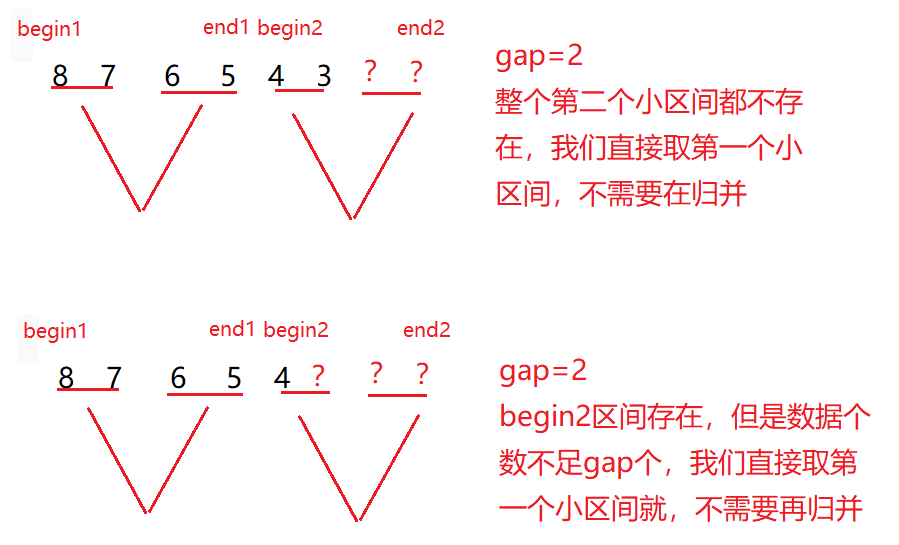void MergeSortNonR(int* a, int n)
{
int* tmp = (int*)malloc(sizeof(int)*n);
if (tmp == NULL)
{
printf("malloc fail\n");
exit(-1);
}

int gap = 1;
while (gap < n)
{
for (int i = 0; i < n; i += 2 * gap)
{
// [i,i+gap-1] [i+gap,i+2*gap-1]
int begin1 = i, end1 = i + gap - 1;
int begin2 = i + gap, end2 = i + 2 * gap - 1;

// 核心思想：end1、begin2、end2都有可能越界
// end1越界 或者 begin2 越界都不需要归并
if (end1 >= n || begin2 >= n)
{
break;
}

// end2 越界，需要归并，修正end2
if (end2 >= n)
{
end2 = n- 1;
}

int index = i;
while (begin1 <= end1 && begin2 <= end2)
{
if (a[begin1] < a[begin2])
{
tmp[index++] = a[begin1++];
}
else
{
tmp[index++] = a[begin2++];
}
}

while (begin1 <= end1)
{
tmp[index++] = a[begin1++];
}

while (begin2 <= end2)
{
tmp[index++] = a[begin2++];
}

// 把归并小区间拷贝回原数组
for (int j = i; j <= end2; ++j)
{
a[j] = tmp[j];
}
}

gap *= 2;
}

free(tmp);
tmp = NULL;
}


①缺点是需要O(N)的空间复杂度，归并排序更多的是解决磁盘外排序的问题

②时间复杂度:O(N*logN)

③空间复杂度:O(N)

# 八、计数排序

1、统计相同元素出现的个数

2、根据统计的结果，将数据拷贝回原数组

①统计相同元素出现的个数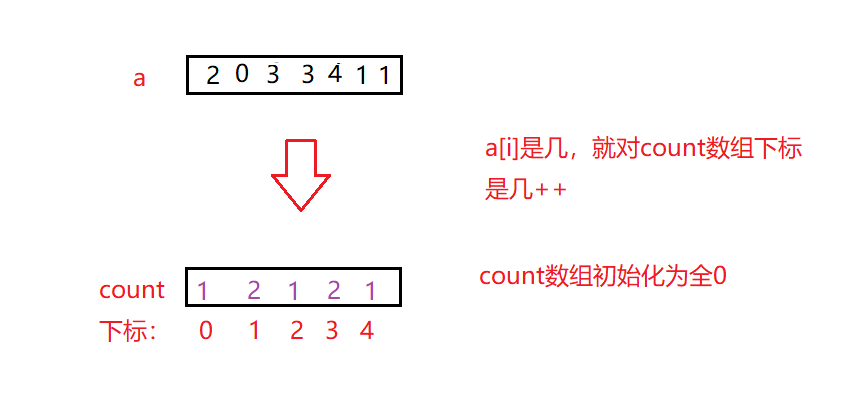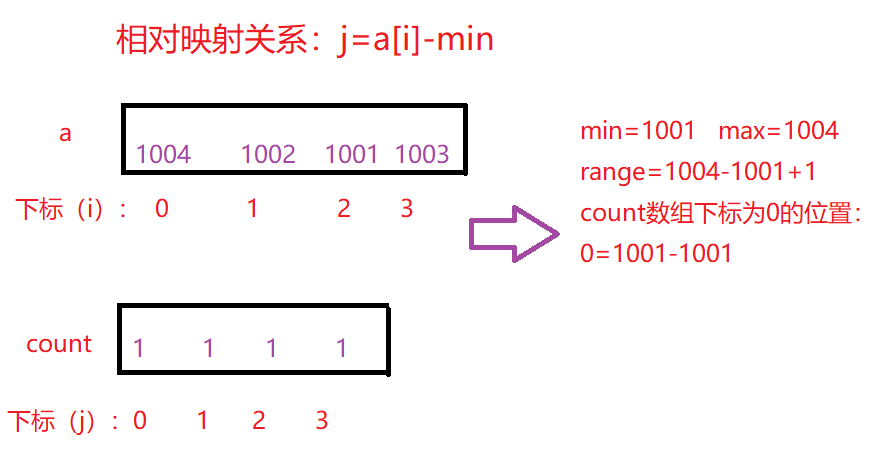②根据count数组的结果，将数据拷贝回a数组

count[j]中数据是几，说明该数出现了几次，是0就不用拷贝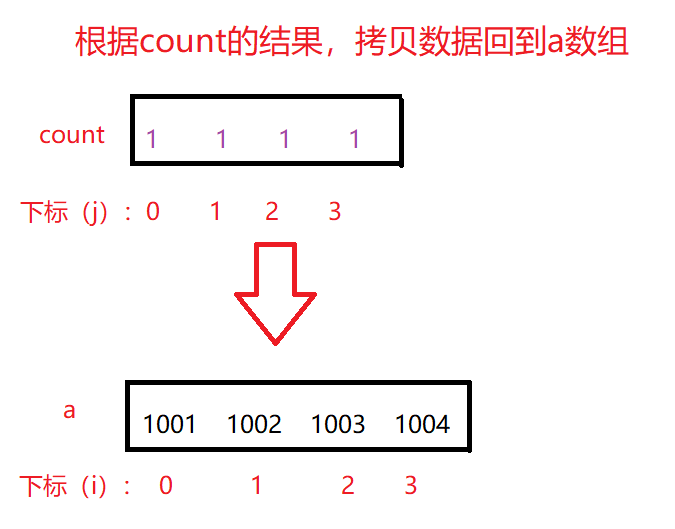代码如下：

void CountSort(int* a, int n)
{
int min = a, max = a;//如果不赋值，min和max就是默认随机值，最好给赋值一个a

for (int i=1;i<n;i++)//修正 找出A数组中的最大值和最小值
{
if (a[i] < min)
{
min=a[i];
}
if (a[i]>max)
{
max=a[i];
}
}
int range = max - min + 1;//控制新开数组的大小，以免空间浪费
int* count = (int*)malloc(sizeof(int) * range);
memset(count,0, sizeof(int) * range);//初始化为全0
if (count==NULL)
{
printf("malloc fail\n");
exit(-1);
}

//1、统计数据个数
for (int i=0;i<n;i++)
{
count[a[i]-min]++;
}
//2、拷贝回A数组
int j = 0;
for (int i=0;i<range;i++)
{
while (count[i]--)
{
a[j++] = i + min;
}
}
free(count);
count = NULL;
}

计数排序总结：

①在数据范围比较集中时，效率很高，但是使用场景很有限，可以排负数，但对于浮点数无能为力

②时间复杂度：O(MAX(N,range))

③空间复杂度：O(range)

# 八大排序的稳定性总结：

08-29
09-06272
10-26428
04-091216
02-095083
01-071445
10-232320
05-042946
10-17655
03-25
01-29517
02-201万+
10-11743
07-175053
03-017501
03-231999
03-142718
03-122391
02-081813

### “相关推荐”对你有帮助么？

•非常没帮助
•没帮助
•一般
•有帮助
•非常有帮助被折叠的  条评论 为什么被折叠?到【灌水乐园】发言Hero 2021

¥2 ¥4 ¥6 ¥10 ¥20余额支付 (余额：-- )扫码支付获取中扫码支付点击重新获取扫码支付1.余额是钱包充值的虚拟货币，按照1:1的比例进行支付金额的抵扣。
2.余额无法直接购买下载，可以购买VIP、C币套餐、付费专栏及课程。余额充值